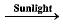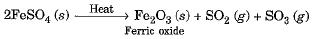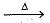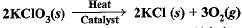Courses

# Chemical Reactions & Equations - 2

## 20 Questions MCQ Test Science Class 10 | Chemical Reactions & Equations - 2

Description
This mock test of Chemical Reactions & Equations - 2 for Class 10 helps you for every Class 10 entrance exam. This contains 20 Multiple Choice Questions for Class 10 Chemical Reactions & Equations - 2 (mcq) to study with solutions a complete question bank. The solved questions answers in this Chemical Reactions & Equations - 2 quiz give you a good mix of easy questions and tough questions. Class 10 students definitely take this Chemical Reactions & Equations - 2 exercise for a better result in the exam. You can find other Chemical Reactions & Equations - 2 extra questions, long questions & short questions for Class 10 on EduRev as well by searching above.
QUESTION: 1

### Mark the incorrect statement :

Solution:

“The statement that substances appearing on the left hand side of the equation are called products” is incorrect.

QUESTION: 2

### 2AgI(s)2Ag(s) + I2(g) The colour of iodine is

Solution:

Iodine is purple in colour.

QUESTION: 3

### What is the number of iron atoms and hydrogen atoms in the balanced equation for the reaction of steam on iron ?

Solution:

The balanced equation for the reaction is
3Fe + 4H2O → Fe3O4 + 4H2

QUESTION: 4

MnO2 + 4HCI→ MnCl2 + H2O + Cl2 The oxidising agent is

Solution:

MnO2 is oxidising agent because it is removing hydrogen from HCI to form Cl2.

QUESTION: 5

The shiny finish to the walls is because of

Solution:

The calcium hydroxide used for white washing the walls reacts with the carbon dioxide (CO2) present in the air to form Calcium carbonate (CaCO3) which gives shiny appearance to the walls.
The chemical reaction occurring during the conversion of slaked lime (calcium hydroxide) to calcium carbonate which gives the shiny appearance to the walls is as follows:
Ca(OH)2 + CO2 → CaCO3 + H2O

QUESTION: 6

Zinc reacts with silver nitrate to form which compounds?

Solution:

Zn + 2AgNO3→ Zn(NO3)2 + 2Ag

QUESTION: 7

On heating ferrous sulphate crystals, we obtain

Solution:

The reaction takes place as underQUESTION: 8

The brown gas evolved on heating of copper nitrate is

Solution:

2Cu(NO3)22CuO + 4NO2 + O2

QUESTION: 9

Which of the following are exothermic processes ?
(i) Reaction of water with quick lime
(ii) Dilution of an acid
(iii) Evaporation of water
(iv) Sublimation of camphor (crystals)

Solution:

Heat is evolved only when water reacts with quick lime and when the acid is diluted with water.

QUESTION: 10

In which of the following chemical equations, the abbreviations represent the correct states of the reactants and products involved at reaction temperature?

Solution:

As we know, H2 and O2 are present in the form of gas in atmosphere and H2O as liquid.

QUESTION: 11

The following reaction is an example of a
(i) Displacement reaction.
(ii) Combination reaction.
(iii) Redox reaction.
(iv) Neutralisation reaction.
4NH3 (g) + 5O2(g) → 4NO(g) + 6H2O(g)

Solution:

In NH3, H has been displaced by O. Thus, it is a displacement reaction. Also NH3 has been oxidised to NO and O2 has been reduced to H2O. This is a redox reaction also.

QUESTION: 12

Barium chloride on reacting with ammonium sulphate forms barium sulphate and ammonium chloride. Which of the following correctly represents the type of the reaction involved
(i) Displacement reaction
(ii) Precipitation reaction
(iii) Combination reaction
(iv) Double displacement reaction

Solution:

BaCl2​ + (NH4​)2​SO4​ →  BaSO4​ + 2NH4​Cl
Above reaction is a double displacement reaction as ions (both cations and anions) of the reacting molecules are exchanged. Barium ion (Ba2+) combines with sulphate ion (SO42−​) to form Barium sulphate (BaSO4​) and ammonium ion (NH4+​) combines with chloride ion(Cl) ion to form ammonium chloride(NH4​Cl).
The above reaction is also classified as a precipitation reaction because barium and sulphate ions combine to form precipitates of barium sulphate.
Ba2+ + SO42−​→ BaSO4​(s)

QUESTION: 13

Which of the following is (are) an endothermic process(es) ?
(i) Dilution of sulphuric acid
(ii) Sublimation of dry ice
(iii) Condensation of water vapours
(iv) Evaporation of water

Solution:

Heat is absorbed when dry ice sublimes and water evaporates. Therefore, cooling is produced.

QUESTION: 14

The following reaction is used for the preparation of oxygen gas in the laboratoryWhich of the following statement (s) is (are) correct about the reaction ?

Solution:

Clearly, It is a decomposition reaction and it requires heat to get decomposed into KCl and O2.

QUESTION: 15

Solid calcium oxide reacts vigorously with water to form calcium hydroxide accompanied by liberation of heat. This process is called slaking of lime. Calcium hydroxide dissolves in water to form its solution called lime water. Which among the following is (are) true about slaking of lime and the solution formed ?
(i) It is an endothermic reaction.
(ii) It is an exothermic reaction.
(iii) The pH of the resulting solution will be more than seven.
(iv) The pH of the resulting solution will be less than seven.

Solution:

Quick lime(CaO) combines with water to form calcium hydroxide (Ca(OH)2). It is an exothermic reaction as heat is produced in the reaction.
CaO + H2O → Ca(OH)2 + heat
On  adding excess of calcium hydroxide in water, a saturated solution of calcium hydroxide is formed which is called lime water. Lime water is basic in nature and therefore has a pH above 7.

QUESTION: 16

Which of the following gases can be used for storage of fresh sample of an oil for a long time?

Solution:

Nitrogen or helium can be used for storage of fresh sample of oil for long time. Helium and Nitrogen both the gases provide inert atmosphere.

QUESTION: 17

Which among the following is (are) double displacement reaction(s) ?
(i) Pb + CuCl2 → PbCl2 + Cu
(ii) Na2SO4 + BaCl2 → BaSO4 + 2NaCl
(iii) C + O2 → CO
(iv) CH4 + 2O2 →CO2 + 2H2O

Solution:

The anions and cations are exchanged in the reaction (ii) only.

QUESTION: 18

Electrolysis of water is a decomposition reaction. The molar ratio of hydrogen and oxygen gases liberated during electrolysis of water is

Solution:

The water decomposes during electrolysis to form hydrogen and oxygen gases in the ratio 2:1 by volume.

QUESTION: 19

Three beakers labelled as A, B and C each containing 25 ml of water were taken. A small amount of NaOH, anhydrous CuSO4 and NaCl were added to the beakers A, B and C respectively. It was observed that there was an increase in the temperature of the solutions contained in beakers A and B, whereas in case of beaker C, the temperature of the solution falls. Which one of the following statement(s) is (are) correct ?
(i) In beakers A and B, exothermic process has occurred
(ii) In beakers A and B, endothermic process has occurred
(iii) In beaker C exothermic process has occurred
(iv) In beaker C endothermic process has occurred

Solution:

Increase of temperature means exothermic process and fall of temperature means endothermic process.

QUESTION: 20

Which one of the following processes involve chemical reactions ?

Solution:

Copper wire on heating in presence of air at high temperature forms copper oxide, which is a chemical reaction. Processes in (a), (b) and (c) do not involve any chemical change.